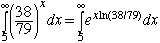Subject: Fwd: convergence Hi, I am a high school senior and I need help stugying for a final. I am stuck on one of the questions on my review sheet. Does the improper integral from 5 to infinity of (38/97)x converge or diverge? If it converges I also need to know how to find the approximate value accurate to .01 of its actual value. Thanks Hi, In your calculus class you have probably spent quite a bit of time on THE exponential function ex and not much time on other exponential functions like 7x or (38/97)x. The reason is that any exponential function ax can be written with base e as follows: Suppose that y = ax then ln(y) = ln(ax) = x ln(a) and hence y = eln(y) = ex ln(a). In your problem (38/97)x = ex ln(38/79) and hence findrecalling that ln(38/79) = ln(38) - ln(79). Cheers, Harley Go to Math Central